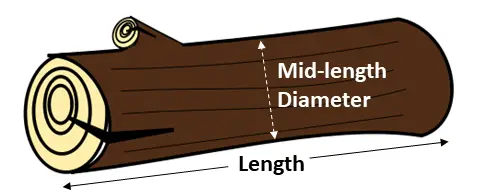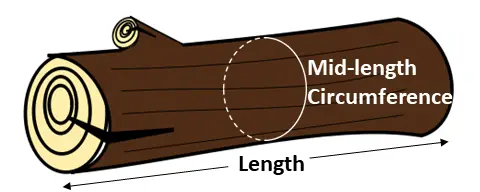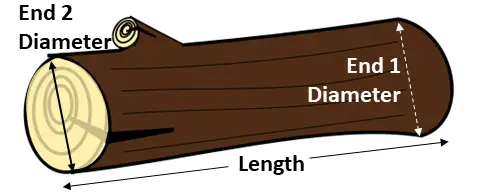# Log Weight Calculator for Any Wood Species

This log weight calculator will estimate the weight and density of any species and any size green (freshly cut) log.

For other wood weight and volume estimations, check out:

## LOG WEIGHT CALCULATOR

*The densities used in this calculator are derived from the Green Log Weight Chart created by the Tree Care Industry Association. This is a handy chart to carry around if you are a logger or someone in the forestry industry.

## How To Use This Calculator

Weight = Density * Volume, so first we will select the proper density by selecting the tree species.

Each species of wood has a different density and therefore different total log weight. The most common tree in the USA is the red maple so that option is pre-selected.

Next, determine how you will measure the log so we can find the volume.  Since Volume of a Cylinder = Cross-Sectional Area * Length, we first need to find the cross sectional area of the log. We can do that by measuring one of these three ways:

• The first option is to approximate the mid-length diameter of the log in inches. This is the diameter of the log at the mid-point. Since you can’t directly measure this without cutting the log in half, an approximation is needed.• The second option is to measure the circumference at the mid-point of the log in inches. This would involve, for example, wrapping a flexible measuring tape around the log at the mid-point.• The third and most accurate way is to measure the diameters of each end of the log in inches and plug those into the calculator.Finally, enter the length of the log in feet and inches. If you have more than one of these logs, enter that into the calculator as well to calculate total weight.